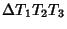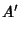## Tangential TriangleThe Triangleformed by the lines tangent to the Circumcircle of a given Triangleat its Vertices. It is the Pedal Triangle ofwith the Circumcenter as the Pedal Point. The Trilinear Coordinates of the Vertices of the tangential triangle areThe Contact Triangle and tangential triangle are perspective from the Gergonne Point.